## 3.3.1 Non-Equilibrium Ensemble Average

We employ the standard device for obtaining a non-equilibrium state. At time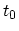, prior to which the system is assumed to be in thermodynamic equilibrium with a reservoir, the system is exposed to a disturbance represented by the contribution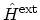to the HAMILTONian. The external perturbation can for instance be a time varying electric field, a light excitation pulse, and so forth. The total HAMILTONian is thus given by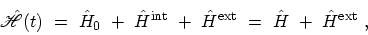(3.16)

where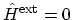for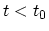. One is not restricted to using the statistical equilibrium state at times prior toas the initial condition. As shown by , a non-equilibrium situation can be maintained through contact with a reservoir. A discussion of the coupling of a system to a reservoir has been studied in .

Non-equilibrium statistical mechanics is concerned with calculating average values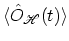of physical observables for times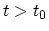. Given the density operator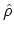, the average of any operator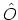is then defined as (C.8)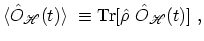(3.17)

where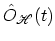is an operator in the HEISENBERG picture.

The non-equilibrium GREEN's function can be defined as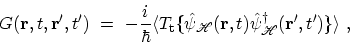(3.18)

where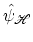is the field operator in the HEISENBERG picture evolving with the HAMILTONian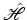defined in (3.16) and the bracket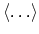is the statistical average with the density operator defined in (3.17).

One can evaluate GREEN's functions by using WICK's theorem, which enables us to decompose many-particle GREEN's functions into sums and products of single-particle GREEN's functions (see Section 3.4.1). The restriction of the WICK theorem necessitates that the field operators and the density operator have to be represented in the interaction picture, or equivalently, their time evolution is governed by the non-interacting HAMILTONian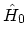. The contour-ordered GREEN's function, which is introduced next, provides a suitable framework for this purpose.

M. Pourfath: Numerical Study of Quantum Transport in Carbon Nanotube-Based Transistors